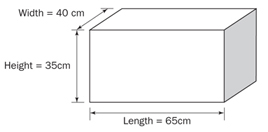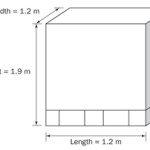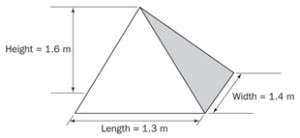Austwide Freight# HOW TO MEASURE YOUR FREIGHT

Cubic conversion is the term given to measlocationng the size of a freight consignment. Large, lightweight shipments may take up more space on the trailer than heavy weight freight items, so it is important the size of freight is taken into consideration when calculating freight costs.

The AustWIDE Freight Standard Cubic Conversion is 1 cubic metre = 333 kilograms.

##### EXAMPLE 1. CUBING A CARTON

Actual Weight = 15kg
Multiply the height x length x width = m3 (cubic measurement)
0.35 x 0.65 x 0.40 = 0.091m3

To convert 0.091m3 to a weight in kilos
multiply 0.091m3 x 333 (standard cubic conversion) = 22.75kg
Therefore 22.75kg is greater than the actual weight of 15kg##### EXAMPLE 1. CUBING AN ODD SHAPED ITEM

Actual Weight = 580kg
multiply the height x length x width = m3 (cubic measurement)
1.60 x 1.30 x 1.40 = 2.912m3

To convert 2.912m3 to a weight in kilos
multiply 2.912m3 x 333 (standard cubic conversion) = 728kg
Therefore 728kg is greater than the actual weight of 580kg

##### EXAMPLE 2. CUBING A PALLET OR SKID

Actual Weight = 450kg
multiply the height x length x width = m3 (cubic measurement)
1.90 x 1.20 x 1.20 = 2.736m3

To convert 2.736m3 to a weight in kilos
multiply 2.736m3 x 333 (standard cubic conversion) = 684kg
Therefore 684kg is greater than the actual weight of 450kg